# 10 Colt Single-Row Functions

This chapter provides a reference to Colt single-row functions included in Oracle Continuous Query Language (Oracle CQL). Colt single-row functions are based on the Colt open source libraries for high performance scientific and technical computing.

This chapter includes the following section:

## 10.1 Introduction to Oracle CQLBuilt-In Single-Row Colt Functions

Table 10-1 lists the built-in single-row Colt functions that Oracle CQL provides.

Table 10-1 Oracle CQL Built-in Single-Row Colt-Based Functions

Colt Package Function

`cern.jet.math.Arithmetic`

A set of basic polynomials, rounding, and calculus functions.

• `binomial`

• `binomial1`

• `ceil`

• `factorial`

• `floor`

• `log`

• `log2`

• `log10`

• `logFactorial`

• `longFactorial`

• `stirlingCorrection`

`cern.jet.math.Bessel`

A set of Bessel functions.

• `i0`

• `i0e`

• `i1`

• `i1e`

• `j0`

• `j1`

• `jn`

• `k0`

• `k0e`

• `k1`

• `k1e`

• `kn`

• `y0`

• `y1`

• `yn`

`cern.jet.random.engine.RandomSeedTable`

A table with good seeds for pseudo-random number generators. Each sequence in this table has a period of 10**9 numbers.

• `getSeedAtRowColumn`

`cern.jet.stat.Gamma`

A set of Gamma and Beta functions.

• `beta`

• `gamma`

• `incompleteBeta`

• `incompleteGamma`

• `incompleteGammaComplement`

• `logGamma`

`cern.jet.stat.Probability`

A set of probability distributions.

• `beta1`

• `betaComplemented`

• `binomial2`

• `binomialComplemented`

• `chiSquare`

• `chiSquareComplemented`

• `errorFunction`

• `errorFunctionComplemented`

• `gamma1`

• `gammaComplemented`

• `negativeBinomial`

• `negativeBinomialComplemented`

• `normal`

• `normal1`

• `normalInverse`

• `poisson`

• `poissonComplemented`

• `studentT`

• `studentTInverse`

`cern.colt.bitvector.QuickBitVector`

A set of non polymorphic, non bounds checking, low level bit-vector functions.

• `bitMaskWithBitsSetFromTo`

• `leastSignificantBit`

• `mostSignificantBit`

`cern.colt.map.HashFunctions`

A set of hash functions.

• `hash`

• `hash1`

• `hash2`

• `hash3`

Note:

Built-in function names are case sensitive and you must use them in the case shown (in lower case).

Note:

In stream input examples, lines beginning with `h` (such as `h 3800`) are heartbeat input tuples. These inform Oracle Event Processing that no further input will have a timestamp lesser than the heartbeat value.

## beta

Syntax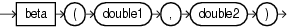Purpose

`beta` is based on `cern.jet.stat.Gamma`. It returns the beta function (see Figure 10-1) of the input arguments as a `double`.

Figure 10-1 cern.jet.stat.Gamma beta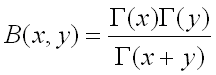This function takes the following arguments:

• `double1`: the `x` value.

• `double2`: the `y` value.

For more information, see `http://dsd.lbl.gov/~hoschek/colt/api/cern/jet/stat/Gamma.html#beta(double,%20double)`.

Examples

Consider the query `qColt28` in Example 10-1. Given the data stream `SColtFunc` with schema `(c1 integer, c2 double, c3 bigint)` in Example 10-2, the query returns the relation in Example 10-3.

Example 10-1 beta Function Query

```<query id="qColt28"><![CDATA[
select beta(c2,c2) from SColtFunc
]]></query>
```

Example 10-2 beta Function Stream Input

```Timestamp   Tuple
10        1,0.5,8
1000        4,0.7,6
1200        3,0.89,12
2000        8,0.4,4
```

Example 10-3 beta Function Relation Output

```Timestamp   Tuple Kind  Tuple
10:       +           3.1415927
1000:       +           1.899038
1200:       +           1.251922
2000:       +           4.226169
```

## beta1

Syntax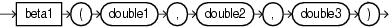Purpose

`beta1` is based on `cern.jet.stat.Probability`. It returns the area `P(x)` from `0` to `x` under the beta density function (see Figure 10-2) as a `double`.

Figure 10-2 cern.jet.stat.Probability beta1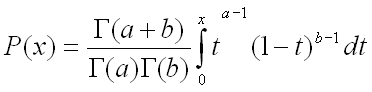This function takes the following arguments:

• `double1`: the alpha parameter of the beta distribution `a`.

• `double2`: the beta parameter of the beta distribution `b`.

• `double3`: the integration end point `x`.

For more information, see `http://dsd.lbl.gov/~hoschek/colt/api/cern/jet/stat/Probability.html#beta(double,%20double,%20double)`.

Examples

Consider the query `qColt35` in Example 10-4. Given the data stream `SColtFunc` with schema `(c1 integer, c2 double, c3 bigint)` in Example 10-5, the query returns the relation in Example 10-6.

Example 10-4 beta1 Function Query

```<query id="qColt35"><![CDATA[
select beta1(c2,c2,c2) from SColtFunc
]]></query>
```

Example 10-5 beta1 Function Stream Input

```Timestamp   Tuple
10        1,0.5,8
1000        4,0.7,6
1200        3,0.89,12
2000        8,0.4,4
```

Example 10-6 beta1 Function Relation Output

```Timestamp   Tuple Kind  Tuple
10:       +           0.5
1000:       +           0.66235894
1200:       +           0.873397
2000:       +           0.44519535
```

## betaComplemented

Syntax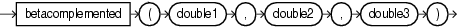Purpose

`betaComplemented` is based on `cern.jet.stat.Probability`. It returns the area under the right hand tail (from `x` to infinity) of the beta density function (see Figure 10-2) as a `double`.

This function takes the following arguments:

• `double1`: the alpha parameter of the beta distribution `a`.

• `double2`: the beta parameter of the beta distribution `b`.

• `double3`: the integration end point `x`.

• "incompleteBeta"

• `http://dsd.lbl.gov/~hoschek/colt/api/cern/jet/stat/Probability.html#betaComplemented(double,%20double,%20double)`

Examples

Consider the query `qColt37` in Example 10-7. Given the data stream `SColtFunc` with schema `(c1 integer, c2 double, c3 bigint)` in Example 10-8, the query returns the relation in Example 10-9.

Example 10-7 betaComplemented Function Query

```<query id="qColt37"><![CDATA[
select betaComplemented(c2,c2,c2) from SColtFunc
]]></query>
```

Example 10-8 betaComplemented Function Stream Input

```Timestamp   Tuple
10        1,0.5,8
1000        4,0.7,6
1200        3,0.89,12
2000        8,0.4,4
```

Example 10-9 betaComplemented Function Relation Output

```Timestamp   Tuple Kind  Tuple
10:       +           0.5
1000:       +           0.66235894
1200:       +           0.873397
2000:       +           0.44519535
```

## binomial

Syntax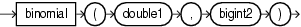Purpose

`binomial` is based on `cern.jet.math.Arithmetic`. It returns the binomial coefficient `n` over `k` (see Figure 10-3) as a `double`.

Figure 10-3 Definition of binomial coefficient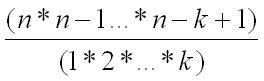This function takes the following arguments:

• `double1`: the `n` value.

• `long2`: the `k` value.

Table 10-2 lists the `binomial` function return values for various values of `k`.

Table 10-2 cern.jet.math.Arithmetic binomial Return Values

Arguments Return Value

`k` < 0

0

`k` = 0

1

`k` = 1

`n`

Any other value of `k`

Computed binomial coefficient as given in Figure 10-3.

For more information, see `http://dsd.lbl.gov/~hoschek/colt/api/cern/jet/math/Arithmetic.html#binomial(double,%20long)`.

Examples

Consider the query `qColt6` in Example 10-10. Given the data stream `SColtFunc` with schema `(c1 integer, c2 double, c3 long)` in Example 10-11, the query returns the relation in Example 10-12.

Example 10-10 binomial Function Query

```<query id="qColt6"><![CDATA[
select binomial(c2,c3) from SColtFunc
]]></query>
```

Example 10-11 binomial Function Stream Input

```Timestamp   Tuple
10        1,0.5,8
1000        4,0.7,6
1200        3,0.89,12
2000        8,0.4,4
```

Example 10-12 binomial Function Relation Output

```Timestamp   Tuple Kind  Tuple
10:       +           -0.013092041
1000:       +           -0.012374863
1200:       +           -0.0010145549
2000:       +           -0.0416
```

## binomial1

Syntax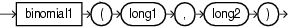Purpose

`binomial1` is based on `cern.jet.math.Arithmetic`. It returns the binomial coefficient `n` over `k` (see Figure 10-3) as a `double`.

This function takes the following arguments:

• `long1`: the `n` value.

• `long2`: the `k` value.

Table 10-3 lists the `BINOMIAL` function return values for various values of `k`.

Table 10-3 cern.jet.math.Arithmetic Binomial1 Return Values

Arguments Return Value

`k` < 0

0

`k` = 0 || `k` = `n`

1

`k` = 1 || `k` = `n`-1

`n`

Any other value of `k`

Computed binomial coefficient as given in Figure 10-3.

For more information, see `http://dsd.lbl.gov/~hoschek/colt/api/cern/jet/math/Arithmetic.html#binomial(long,%20long)`.

Examples

Consider the query `qColt7` in Example 10-13. Given the data stream `SColtFunc` with schema `(c1 integer, c2 float, c3 long)` in Example 10-14, the query returns the relation in Example 10-15.

Example 10-13 binomial1 Function Query

```<query id="qColt7"><![CDATA[
select binomial1(c3,c3) from SColtFunc
]]></query>
```

Example 10-14 binomial1 Function Stream Input

```Timestamp   Tuple
10        1,0.5,8
1000        4,0.7,6
1200        3,0.89,12
2000        8,0.4,4
```

Example 10-15 binomial1 Function Relation Output

```Timestamp   Tuple Kind  Tuple
10:       +           1.0
1000:       +           1.0
1200:       +           1.0
2000:       +           1.0
```

## binomial2

Syntax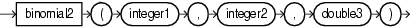Purpose

`binomial2` is based on `cern.jet.stat.Probability`. It returns the sum of the terms 0 through `k` of the binomial probability density (see Figure 10-4) as a `double`.

Figure 10-4 cern.jet.stat.Probability binomial2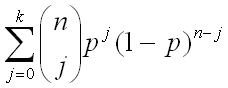This function takes the following arguments (all arguments must be positive):

• `integer1`: the end term `k`.

• `integer2`: the number of trials `n`.

• `double3`: the probability of success `p` in (0.0, 1.0)

For more information, see `http://dsd.lbl.gov/~hoschek/colt/api/cern/jet/stat/Probability.html#binomial(int,%20int,%20double)`.

Examples

Consider the query `qColt34` in Example 10-16. Given the data stream `SColtFunc` with schema `(c1 integer, c2 double, c3 bigint)` in Example 10-17, the query returns the relation in Example 10-18.

Example 10-16 binomial2 Function Query

```<query id="qColt34"><![CDATA[
select binomial2(c1,c1,c2) from SColtFunc
]]></query>
```

Example 10-17 binomial2 Function Stream Input

```Timestamp   Tuple
10        1,0.5,8
1000        4,0.7,6
1200        3,0.89,12
2000        8,0.4,4
```

Example 10-18 binomial2 Function Relation Output

```Timestamp   Tuple Kind  Tuple
10:       +           1.0
1000:       +           1.0
1200:       +           1.0
2000:       +           1.0
```

## binomialComplemented

Syntax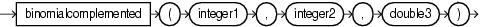Purpose

`binomialComplemented` is based on `cern.jet.stat.Probability`. It returns the sum of the terms `k+1` through `n` of the binomial probability density (see Figure 10-5) as a `double`.

Figure 10-5 cern.jet.stat.Probability binomialComplemented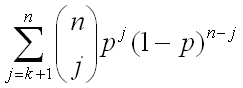This function takes the following arguments (all arguments must be positive):

• `integer1`: the end term `k`.

• `integer2`: the number of trials `n`.

• `double3`: the probability of success `p` in (0.0, 1.0)

For more information, see `http://dsd.lbl.gov/~hoschek/colt/api/cern/jet/stat/Probability.html#binomialComplemented(int,%20int,%20double)`.

Examples

Consider the query `qColt38` in Example 10-19. Given the data stream `SColtFunc` with schema `(integer, c2 double, c3 bigint)` in Example 10-20, the query returns the relation in Example 10-21.

Example 10-19 binomialComplemented Function Query

```<query id="qColt38"><![CDATA[
select binomialComplemented(c1,c1,c2) from SColtFunc
]]></query>
```

Example 10-20 binomialComplemented Function Stream Input

```Timestamp   Tuple
10        1,0.5,8
1000        4,0.7,6
1200        3,0.89,12
2000        8,0.4,4
```

Example 10-21 binomialComplemented Function Relation Output

```Timestamp   Tuple Kind  Tuple
10:       +           0.0
1000:       +           0.0
1200:       +           0.0
2000:       +           0.0
```

Syntax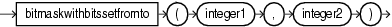Purpose

`bitMaskWithBitsSetFromTo` is based on `cern.colt.bitvector.QuickBitVector`. It returns a 64-bit wide bit mask as a `long` with bits in the specified range set to 1 and all other bits set to 0.

This function takes the following arguments:

• `integer1`: the `from` value; index of the start bit (inclusive).

• `integer2`: the `to` value; index of the end bit (inclusive).

Precondition (not checked): `to` - `from` + 1 >= 0 && `to` - `from` + 1 <= 64.

If `to` - `from` + 1 = 0 then returns a bit mask with all bits set to 0.

Examples

Consider the query `qColt53` in Example 10-22. Given the data stream `SColtFunc` with schema `(c1 integer, c2 float, c3 bigint)` in Example 10-23, the query returns the relation in Example 10-24.

```<query id="qColt53"><![CDATA[
]]></query>
```

Example 10-23 bitMaskWithBitsSetFromTo Function Stream Input

```Timestamp   Tuple
10        1,0.5,8
1000        4,0.7,6
1200        3,0.89,12
2000        8,0.4,4
```

Example 10-24 bitMaskWithBitsSetFromTo Function Relation Output

```Timestamp   Tuple Kind  Tuple
10:       +           2
1000:       +           16
1200:       +           8
2000:       +           256
```

## ceil

Syntax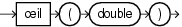Purpose

`ceil` is based on `cern.jet.math.Arithmetic`. It returns the smallest `long` greater than or equal to its `double` argument.

This method is safer than using `(float) java.lang.Math.ceil(long)` because of possible rounding error.

• `http://dsd.lbl.gov/~hoschek/colt/api/cern/jet/math/Arithmetic.html#ceil(double)`

• "ceil1"

Examples

Consider the query `qColt1` in Example 10-25. Given the data stream `SColtFunc` with schema `(c1 integer, c2 double, c3 bigint)` in Example 10-26, the query returns the relation in Example 10-27.

Example 10-25 ceil Function Query

```<query id="qColt1"><![CDATA[
select ceil(c2) from SColtFunc
]]></query>
```

Example 10-26 ceil Function Stream Input

```Timestamp   Tuple
10        1,0.5,8
1000        4,0.7,6
1200        3,0.89,12
2000        8,0.4,4
```

Example 10-27 ceil Function Relation Output

```Timestamp   Tuple Kind  Tuple
10:       +           1
1000:       +           1
1200:       +           1
2000:       +           1
```

## chiSquare

SyntaxPurpose

`chiSquare` is based on `cern.jet.stat.Probability`. It returns the area under the left hand tail (from 0 to `x`) of the Chi square probability density function with `v` degrees of freedom (see Figure 10-6) as a `double`.

Figure 10-6 cern.jet.stat.Probability chiSquare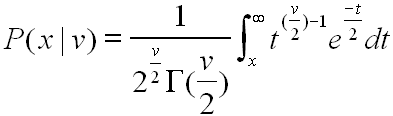This function takes the following arguments (all arguments must be positive):

• `double1`: the degrees of freedom `v`.

• `double2`: the integration end point `x`.

For more information, see `http://dsd.lbl.gov/~hoschek/colt/api/cern/jet/stat/Probability.html#chiSquare(double,%20double)`.

Examples

Consider the query `qColt39` in Example 10-28. Given the data stream `SColtFunc` with schema `(c1 integer, c2 double, c3 bigint)` in Example 10-29, the query returns the relation in Example 10-30.

Example 10-28 chiSquare Function Query

```<query id="qColt39"><![CDATA[
select chiSquare(c2,c2) from SColtFunc
]]></query>
```

Example 10-29 chiSquare Function Stream Input

```Timestamp   Tuple
10        1,0.5,8
1000        4,0.7,6
1200        3,0.89,12
2000        8,0.4,4
```

Example 10-30 chiSquare Function Relation Output

```Timestamp   Tuple Kind  Tuple
10:       +           0.0
1000:       +           0.0
1200:       +           0.0
2000:       +           0.0
```

## chiSquareComplemented

Syntax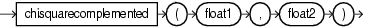Purpose

`chiSquareComplemented` is based on `cern.jet.stat.Probability`. It returns the area under the right hand tail (from `x` to infinity) of the Chi square probability density function with `v` degrees of freedom (see Figure 10-6) as a `double`.

This function takes the following arguments (all arguments must be positive):

• `double1`: the degrees of freedom `v`.

• `double2`: the integration end point `x`.

For more information, see `http://dsd.lbl.gov/~hoschek/colt/api/cern/jet/stat/Probability.html#chiSquareComplemented(double,%20double)`.

Examples

Consider the query `qColt40` in Example 10-31. Given the data stream `SColtFunc` with schema `(c1 integer, c2 double, c3 bigint)` in Example 10-32, the query returns the relation in Example 10-33.

Example 10-31 chiSquareComplemented Function Query

```<query id="qColt40"><![CDATA[
select chiSquareComplemented(c2,c2) from SColtFunc
]]></query>
```

Example 10-32 chiSquareComplemented Function Stream Input

```Timestamp   Tuple
10        1,0.5,8
1000        4,0.7,6
1200        3,0.89,12
2000        8,0.4,4
```

Example 10-33 chiSquareComplemented Function Relation Output

```Timestamp   Tuple Kind  Tuple
10:       +           0.0
1000:       +           0.0
1200:       +           0.0
2000:       +           0.0
```

## errorFunction

Syntax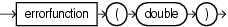Purpose

`errorFunction` is based on `cern.jet.stat.Probability`. It returns the error function of the normal distribution of the `double` argument as a `double`, using the integral that Figure 10-7 shows.

Figure 10-7 cern.jet.stat.Probability errorFunction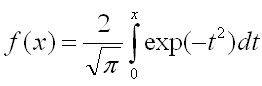For more information, see `http://dsd.lbl.gov/~hoschek/colt/api/cern/jet/stat/Probability.html#errorFunction(double)`.

Examples

Consider the query `qColt41` in Example 10-34. Given the data stream `SColtFunc` with schema `(c1 integer, c2 double, c3 bigint)` in Example 10-35, the query returns the relation in Example 10-36.

Example 10-34 errorFunction Function Query

```<query id="qColt41"><![CDATA[
select errorFunction(c2) from SColtFunc
]]></query>
```

Example 10-35 errorFunction Function Stream Input

```Timestamp   Tuple
10        1,0.5,8
1000        4,0.7,6
1200        3,0.89,12
2000        8,0.4,4
```

Example 10-36 errorFunction Function Relation Output

```Timestamp   Tuple Kind  Tuple
10:       +           0.5204999
1000:       +           0.6778012
1200:       +           0.79184324
2000:       +           0.42839235
```

## errorFunctionComplemented

SyntaxPurpose

`errorFunctionComplemented` is based on `cern.jet.stat.Probability`. It returns the complementary error function of the normal distribution of the `double` argument as a `double`, using the integral that Figure 10-8 shows.

Figure 10-8 cern.jet.stat.Probability errorfunctioncompelemented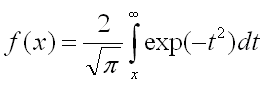For more information, see `http://dsd.lbl.gov/~hoschek/colt/api/cern/jet/stat/Probability.html#errorFunctionComplemented(double)`.

Examples

Consider the query `qColt42` in Example 10-37. Given the data stream `SColtFunc` with schema `(c1 integer, c2 double, c3 bigint)` in Example 10-38, the query returns the relation in Example 10-39.

Example 10-37 errorFunctionComplemented Function Query

```<query id="qColt42"><![CDATA[
select errorFunctionComplemented(c2) from SColtFunc
]]></query>
```

Example 10-38 errorFunctionComplemented Function Stream Input

```Timestamp   Tuple
10        1,0.5,8
1000        4,0.7,6
1200        3,0.89,12
2000        8,0.4,4
```

Example 10-39 errorFunctionComplemented Function Relation Output

```Timestamp   Tuple Kind  Tuple
10:       +           0.47950011
1000:       +           0.3221988
1200:       +           0.20815676
2000:       +           0.57160765
```

## factorial

Syntax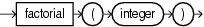Purpose

`factorial` is based on `cern.jet.math.Arithmetic`. It returns the factorial of the positive `integer` argument as a `double`.

For more information, see `http://dsd.lbl.gov/~hoschek/colt/api/cern/jet/math/Arithmetic.html#factorial(int)`.

Examples

Consider the query `qColt8` in Example 10-40. Given the data stream `SColtFunc` with schema `(c1 integer, c2 float, c3 bigint)` in Example 10-41, the query returns the relation in Example 10-42.

Example 10-40 factorial Function Query

```<query id="qColt8"><![CDATA[
select factorial(c1) from SColtFunc
]]></query>
```

Example 10-41 factorial Function Stream Input

```Timestamp   Tuple
10        1,0.5,8
1000        4,0.7,6
1200        3,0.89,12
2000        8,0.4,4
```

Example 10-42 factorial Function Relation Output

```Timestamp   Tuple Kind  Tuple
10:       +               1.0
1000:       +              24.0
1200:       +               6.0
2000:       +           40320.0
```

## floor

Syntax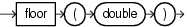Purpose

`floor` is based on `cern.jet.math.Arithmetic`. It returns the largest `long` value less than or equal to the `double` argument.

This method is safer than using `(double) java.lang.Math.floor(double)` because of possible rounding error.

• `http://dsd.lbl.gov/~hoschek/colt/api/cern/jet/math/Arithmetic.html#floor(double)`

• "floor1"

Examples

Consider the query `qColt2` in Example 10-43. Given the data stream `SColtFunc` with schema `(c1 integer, c2 double, c3 bigint)` in Example 10-44, the query returns the relation in Example 10-45.

Example 10-43 floor Function Query

```<query id="qColt2"><![CDATA[
select floor(c2) from SColtFunc
]]></query>
```

Example 10-44 floor Function Stream Input

```Timestamp   Tuple
10        1,0.5,8
1000        4,0.7,6
1200        3,0.89,12
2000        8,0.4,4
```

Example 10-45 floor Function Relation Output

```Timestamp   Tuple Kind  Tuple
10:       +           0
1000:       +           0
1200:       +           0
2000:       +           0
```

## gamma

Syntax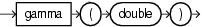Purpose

`gamma` is based on `cern.jet.stat.Gamma`. It returns the Gamma function of the `double` argument as a `double`.

For more information, see `http://dsd.lbl.gov/~hoschek/colt/api/cern/jet/stat/Gamma.html#gamma(double)`.

Examples

Consider the query `qColt29` in Example 10-46. Given the data stream `SColtFunc` with schema `(c1 integer, c2 double, c3 bigint)` in Example 10-47, the query returns the relation in Example 10-48.

Example 10-46 gamma Function Query

```<query id="qColt29"><![CDATA[
select gamma(c2) from SColtFunc
]]></query>
```

Example 10-47 gamma Function Stream Input

```Timestamp   Tuple
10        1,0.5,8
1000        4,0.7,6
1200        3,0.89,12
2000        8,0.4,4
```

Example 10-48 gamma Function Relation Output

```Timestamp   Tuple Kind  Tuple
10:       +           1.7724539
1000:       +           1.2980554
1200:       +           1.0768307
2000:       +           2.2181594
```

## gamma1

Syntax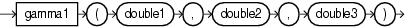Purpose

`gamma1` is based on `cern.jet.stat.Probability`. It returns the integral from zero to `x` of the gamma probability density function (see Figure 10-9) as a `double`.

Figure 10-9 cern.jet.stat.Probability gamma1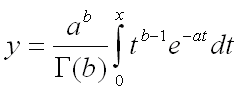This function takes the following arguments:

• `double1`: the gamma distribution alpha value `a`

• `double2`: the gamma distribution beta or lambda value `b`

• `double3`: the integration end point `x`

For more information, see `http://dsd.lbl.gov/~hoschek/colt/api/cern/jet/stat/Probability.html#gamma(double,%20double,%20double)`.

Examples

Consider the query `qColt36` in Example 10-49. Given the data stream `SColtFunc` with schema `(c1 integer, c2 double, c3 bigint)` in Example 10-50, the query returns the relation in Example 10-51.

Example 10-49 gamma1 Function Query

```<query id="qColt36"><![CDATA[
select gamma1(c2,c2,c2) from SColtFunc
]]></query>
```

Example 10-50 gamma1 Function Stream Input

```Timestamp   Tuple
10        1,0.5,8
1000        4,0.7,6
1200        3,0.89,12
2000        8,0.4,4
```

Example 10-51 gamma1 Function Relation Output

```Timestamp   Tuple Kind  Tuple
10:       +           0.5204999
1000:       +           0.55171627
1200:       +           0.59975785
2000:       +           0.51785487
```

## gammaComplemented

Syntax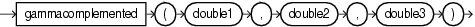Purpose

`gammaComplemented` is based on `cern.jet.stat.Probability`. It returns the integral from `x` to infinity of the gamma probability density function (see Figure 10-10) as a `double`.

Figure 10-10 cern.jet.stat.Probability gammaComplemented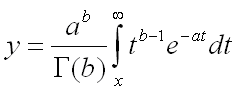This function takes the following arguments:

• `double1`: the gamma distribution alpha value `a`

• `double2`: the gamma distribution beta or lambda value `b`

• `double3`: the integration end point `x`

For more information, see `http://dsd.lbl.gov/~hoschek/colt/api/cern/jet/stat/Probability.html#gammaComplemented(double,%20double,%20double)`.

Examples

Consider the query `qColt43` in Example 10-52. Given the data stream `SColtFunc` with schema `(c1 integer, c2 double, c3 bigint)` in Example 10-53, the query returns the relation in Example 10-54.

Example 10-52 gammaComplemented Function Query

```<query id="qColt43"><![CDATA[
select gammaComplemented(c2,c2,c2) from SColtFunc
]]></query>
```

Example 10-53 gammaComplemented Function Stream Input

```Timestamp   Tuple
10        1,0.5,8
1000        4,0.7,6
1200        3,0.89,12
2000        8,0.4,4
```

Example 10-54 gammaComplemented Function Relation Output

```Timestamp   Tuple Kind  Tuple
10:       +           0.47950011
1000:       +           0.44828376
1200:       +           0.40024218
2000:       +           0.48214513
```

## getSeedAtRowColumn

Syntax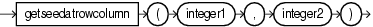Purpose

`getSeedAtRowColumn` is based on `cern.jet.random.engine.RandomSeedTable`. It returns a deterministic seed as an `integer` from a (seemingly gigantic) matrix of predefined seeds.

This function takes the following arguments:

• `integer1`: the `row` value; should (but need not) be in `[0,Integer.MAX_VALUE]`.

• `integer2`: the `column` value; should (but need not) be in `[0,1]`.

For more information, see `http://dsd.lbl.gov/~hoschek/colt/api/cern/jet/random/engine/RandomSeedTable.html#getSeedAtRowColumn(int,%20int)`.

Examples

Consider the query `qColt27` in Example 10-55. Given the data stream `SColtFunc` with schema `(c1 integer, c2 double, c3 bigint)` in Example 10-56, the query returns the relation in Example 10-57.

Example 10-55 getSeedAtRowColumn Function Query

```<query id="qColt27"><![CDATA[
select getSeedAtRowColumn(c1,c1) from SColtFunc
]]></query>
```

Example 10-56 getSeedAtRowColumn Function Stream Input

```Timestamp   Tuple
10        1,0.5,8
1000        4,0.7,6
1200        3,0.89,12
2000        8,0.4,4
```

Example 10-57 getSeedAtRowColumn Function Relation Output

```Timestamp   Tuple Kind  Tuple
10:       +           253987020
1000:       +           1289741558
1200:       +           417696270
2000:       +           350557787
```

## hash

Syntax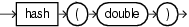Purpose

`hash` is based on `cern.colt.map.HashFunctions`. It returns an `integer` hashcode for the specified `double` value.

For more information, see `http://dsd.lbl.gov/~hoschek/colt/api/cern/colt/map/HashFunctions.html#hash(double)`.

Examples

Consider the query `qColt56` in Example 10-58. Given the data stream `SColtFunc` with schema `(c1 integer, c2 double, c3 bigint)` in Example 10-59, the query returns the relation in Example 10-60.

Example 10-58 hash Function Query

```<query id="qColt56"><![CDATA[
select hash(c2) from SColtFunc
]]></query>
```

Example 10-59 hash Function Stream Input

```Timestamp   Tuple
10        1,0.5,8
1000        4,0.7,6
1200        3,0.89,12
2000        8,0.4,4
```

Example 10-60 hash Function Relation Output

```Timestamp   Tuple Kind  Tuple
10:       +            1071644672
1000:       +            1608935014
1200:       +            2146204385
2000:       +           -1613129319
```

## hash1

Syntax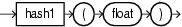Purpose

`hash1` is based on `cern.colt.map.HashFunctions`. It returns an `integer` hashcode for the specified `float` value.

For more information, see `http://dsd.lbl.gov/~hoschek/colt/api/cern/colt/map/HashFunctions.html#hash(float)`.

Examples

Consider the query `qColt57` in Example 10-61. Given the data stream `SColtFunc` with schema `(c1 integer, c2 double, c3 bigint)` in Example 10-62, the query returns the relation in Example 10-63.

Example 10-61 hash1 Function Query

```<query id="qColt57"><![CDATA[
select hash1(c2) from SColtFunc
]]></query>
```

Example 10-62 hash1 Function Stream Input

```Timestamp   Tuple
10        1,0.5,8
1000        4,0.7,6
1200        3,0.89,12
2000        8,0.4,4
```

Example 10-63 hash1 Function Relation Output

```Timestamp   Tuple Kind  Tuple
10:       +           1302214522
1000:       +           1306362078
1200:       +           1309462552
2000:       +           1300047248
```

## hash2

Syntax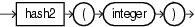Purpose

`hash2` is based on `cern.colt.map.HashFunctions`. It returns an `integer` hashcode for the specified `integer` value.

For more information, see `http://dsd.lbl.gov/~hoschek/colt/api/cern/colt/map/HashFunctions.html#hash(int)`.

Examples

Consider the query `qColt58` in Example 10-64. Given the data stream `SColtFunc` with schema `(c1 integer, c2 double, c3 bigint)` in Example 10-65, the query returns the relation in Example 10-66.

Example 10-64 hash2 Function Query

```<query id="qColt58"><![CDATA[
select hash2(c1) from SColtFunc
]]></query>
```

Example 10-65 hash2 Function Stream Input

```Timestamp   Tuple
10        1,0.5,8
1000        4,0.7,6
1200        3,0.89,12
2000        8,0.4,4
```

Example 10-66 hash2 Function Relation Output

```Timestamp   Tuple Kind  Tuple
10:       +           1
1000:       +           4
1200:       +           3
2000:       +           8
```

## hash3

Syntax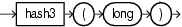Purpose

`hash3` is based on `cern.colt.map.HashFunctions`. It returns an `integer` hashcode for the specified `long` value.

For more information, see `http://dsd.lbl.gov/~hoschek/colt/api/cern/colt/map/HashFunctions.html#hash(long)`.

Examples

Consider the query `qColt59` in Example 10-67. Given the data stream `SColtFunc` with schema `(c1 integer, c2 double, c3 bigint)` in Example 10-68, the query returns the relation in Example 10-69.

Example 10-67 hash3 Function Query

```<query id="qColt59"><![CDATA[
select hash3(c3) from SColtFunc
]]></query>
```

Example 10-68 hash3 Function Stream Input

```Timestamp   Tuple
10        1,0.5,8
1000        4,0.7,6
1200        3,0.89,12
2000        8,0.4,4
```

Example 10-69 hash3 Function Relation Output

```Timestamp   Tuple Kind  Tuple
10:       +           8
1000:       +           6
1200:       +           12
2000:       +           4
```

## i0

Syntax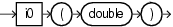Purpose

`i0` is based on `cern.jet.math.Bessel`. It returns the modified Bessel function of order 0 of the `double` argument as a `double`.

The function is defined as `i0(x) = j0(ix)`.

The range is partitioned into the two intervals `[0,8]` and `(8,infinity)`.

• `http://dsd.lbl.gov/~hoschek/colt/api/cern/jet/math/Bessel.html#i0(double)`

• "j0"

Examples

Consider the query `qColt12` in Example 10-70. Given the data stream `SColtFunc` with schema `(c1 integer, c2 double, c3 bigint)` in Example 10-71, the query returns the relation in Example 10-72.

Example 10-70 i0 Function Query

```<query id="qColt12"><![CDATA[
select i0(c2) from SColtFunc
]]></query>
```

Example 10-71 i0 Function Stream Input

```Timestamp   Tuple
10        1,0.5,8
1000        4,0.7,6
1200        3,0.89,12
2000        8,0.4,4
```

Example 10-72 i0 Function Relation Output

```Timestamp   Tuple Kind  Tuple
10:       +           1.0634834
1000:       +           1.126303
1200:       +           1.2080469
2000:       +           1.0404018
```

## i0e

Syntax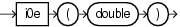Purpose

`i0e` is based on `cern.jet.math.Bessel`. It returns the exponentially scaled modified Bessel function of order 0 of the `double` argument as a `double`.

The function is defined as: `i0e(x) = exp(-|x|) j0(ix)`.

• `http://dsd.lbl.gov/~hoschek/colt/api/cern/jet/math/Bessel.html#i0e(double)`

• "j0"

Examples

Consider the query `qColt13` in Example 10-73. Given the data stream `SColtFunc` with schema `(c1 integer, c2 double, c3 bigint)` in Example 10-74, the query returns the relation in Example 10-75.

Example 10-73 i0e Function Query

```<query id="qColt13"><![CDATA[
select i0e(c2) from SColtFunc
]]></query>
```

Example 10-74 i0e Function Stream Input

```Timestamp   Tuple
10        1,0.5,8
1000        4,0.7,6
1200        3,0.89,12
2000        8,0.4,4
```

Example 10-75 i0e Function Relation Output

```Timestamp   Tuple Kind  Tuple
10:       +           0.64503527
1000:       +           0.55930555
1200:       +           0.4960914
2000:       +           0.6974022
```

## i1

Syntax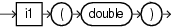Purpose

`i1` is based on `cern.jet.math.Bessel`. It returns the modified Bessel function of order 1 of the `double` argument as a `double`.

The function is defined as: `i1(x) = -i j1(ix)`.

The range is partitioned into the two intervals `[0,8]` and `(8,infinity)`. Chebyshev polynomial expansions are employed in each interval.

• `http://dsd.lbl.gov/~hoschek/colt/api/cern/jet/math/Bessel.html#i1(double)`

• "j1"

Examples

Consider the query `qColt14` in Example 10-76. Given the data stream `SColtFunc` with schema `(c1 integer, c2 double, c3 bigint)` in Example 10-77, the query returns the relation in Example 10-78.

Example 10-76 i1 Function Query

```<query id="qColt14"><![CDATA[
select i1(c2) from SColtFunc
]]></query>
```

Example 10-77 i1 Function Stream Input

```Timestamp   Tuple
10        1,0.5,8
1000        4,0.7,6
1200        3,0.89,12
2000        8,0.4,4
```

Example 10-78 i1 Function Relation Output

```Timestamp   Tuple Kind  Tuple
10:       +           0.2578943
1000:       +           0.37187967
1200:       +           0.49053898
2000:       +           0.20402676
```

## i1e

Syntax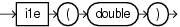Purpose

`i1e` is based on `cern.jet.math.Bessel`. It returns the exponentially scaled modified Bessel function of order 1 of the `double` argument as a `double`.

The function is defined as `i1(x) = -i exp(-|x|) j1(ix)`.

• `http://dsd.lbl.gov/~hoschek/colt/api/cern/jet/math/Bessel.html#i1e(double)`

• "j1"

Examples

Consider the query `qColt15` in Example 10-79. Given the data stream `SColtFunc` with schema `(c1 integer, c2 double, c3 bigint)` in Example 10-80, the query returns the relation in Example 10-81.

Example 10-79 i1e Function Query

```<query id="qColt15"><![CDATA[
select i1e(c2) from SColtFunc
]]></query>
```

Example 10-80 i1e Function Stream Input

```Timestamp   Tuple
10        1,0.5,8
1000        4,0.7,6
1200        3,0.89,12
2000        8,0.4,4
```

Example 10-81 i1e Function Relation Output

```Timestamp   Tuple Kind  Tuple
10:       +           0.1564208
1000:       +           0.18466999
1200:       +           0.20144266
2000:       +           0.13676323
```

## incompleteBeta

Syntax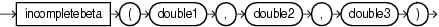Purpose

`incompleteBeta` is based on `cern.jet.stat.Gamma`. It returns the Incomplete Beta Function evaluated from zero to `x` as a `double`.

This function takes the following arguments:

• `double1`: the beta distribution alpha value `a`

• `double2`: the beta distribution beta value `b`

• `double3`: the integration end point `x`

For more information, see `http://dsd.lbl.gov/~hoschek/colt/api/cern/jet/stat/Gamma.html#incompleteBeta(double,%20double,%20double)`.

Examples

Consider the query `qColt30` in Example 10-82. Given the data stream `SColtFunc` with schema `(c1 integer, c2 double, c3 bigint)` in Example 10-83, the query returns the relation in Example 10-84.

Example 10-82 incompleteBeta Function Query

```<query id="qColt30"><![CDATA[
select incompleteBeta(c2,c2,c2) from SColtFunc
]]></query>
```

Example 10-83 incompleteBeta Function Stream Input

```Timestamp   Tuple
10        1,0.5,8
1000        4,0.7,6
1200        3,0.89,12
2000        8,0.4,4
```

Example 10-84 incompleteBeta Function Relation Output

```Timestamp   Tuple Kind  Tuple
10:       +           0.5
1000:       +           0.66235894
1200:       +           0.873397
2000:       +           0.44519535
```

## incompleteGamma

Syntax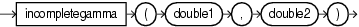Purpose

`incompleteGamma` is based on `cern.jet.stat.Gamma`. It returns the Incomplete Gamma function of the arguments as a `double`.

This function takes the following arguments:

• `double1`: the gamma distribution alpha value `a`.

• `double2`: the integration end point `x`.

For more information, see `http://dsd.lbl.gov/~hoschek/colt/api/cern/jet/stat/Gamma.html#incompleteGamma(double,%20double)`.

Examples

Consider the query `qColt31` in Example 10-85. Given the data stream `SColtFunc` with schema `(c1 integer, c2 double, c3 bigint)` in Example 10-86, the query returns the relation in Example 10-87.

Example 10-85 incompleteGamma Function Query

```<query id="qColt31"><![CDATA[
select incompleteGamma(c2,c2) from SColtFunc
]]></query>
```

Example 10-86 incompleteGamma Function Stream Input

```Timestamp   Tuple
10        1,0.5,8
1000        4,0.7,6
1200        3,0.89,12
2000        8,0.4,4
```

Example 10-87 incompleteGamma Function Relation Output

```Timestamp   Tuple Kind  Tuple
10:       +           0.6826895
1000:       +           0.6565891
1200:       +           0.6397422
2000:       +           0.7014413
```

## incompleteGammaComplement

Syntax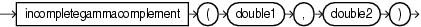Purpose

`incompleteGammaComplement` is based on `cern.jet.stat.Gamma`. It returns the Complemented Incomplete Gamma function of the arguments as a `double`.

This function takes the following arguments:

• `double1`: the gamma distribution alpha value `a`.

• `double2`: the integration start point `x`.

For more information, see `http://dsd.lbl.gov/~hoschek/colt/api/cern/jet/stat/Gamma.html#incompleteGammaComplement(double,%20double)`.

Examples

Consider the query `qColt32` in Example 10-88. Given the data stream `SColtFunc` with schema `(c1 integer, c2 double, c3 bigint)` in Example 10-89, the query returns the relation in Example 10-90.

Example 10-88 incompleteGammaComplement Function Query

```<query id="qColt32"><![CDATA[
select incompleteGammaComplement(c2,c2) from SColtFunc
]]></query>
```

Example 10-89 incompleteGammaComplement Function Stream Input

```Timestamp   Tuple
10        1,0.5,8
1000        4,0.7,6
1200        3,0.89,12
2000        8,0.4,4
```

Example 10-90 incompleteGammaComplement Function Relation Output

```Timestamp   Tuple Kind  Tuple
10:       +           0.3173105
1000:       +           0.34341094
1200:       +           0.3602578
2000:       +           0.29855874
```

## j0

Syntax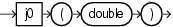Purpose

`j0` is based on `cern.jet.math.Bessel`. It returns the Bessel function of the first kind of order 0 of the `double` argument as a `double`.

For more information, see `http://dsd.lbl.gov/~hoschek/colt/api/cern/jet/math/Bessel.html#j0(double)`.

Examples

Consider the query `qColt16` in Example 10-91. Given the data stream `SColtFunc` with schema `(c1 integer, c2 double, c3 bigint)` in Example 10-92, the query returns the relation in Example 10-93.

Example 10-91 j0 Function Query

```<query id="qColt16"><![CDATA[
select j0(c2) from SColtFunc
]]></query>
```

Example 10-92 j0 Function Stream Input

```Timestamp   Tuple
10        1,0.5,8
1000        4,0.7,6
1200        3,0.89,12
2000        8,0.4,4
```

Example 10-93 j0 Function Relation Output

```Timestamp   Tuple Kind  Tuple
10:       +           0.9384698
1000:       +           0.8812009
1200:       +           0.8115654
2000:       +           0.9603982
```

## j1

Syntax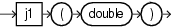Purpose

`j1` is based on `cern.jet.math.Bessel`. It returns the Bessel function of the first kind of order 1 of the `double` argument as a `double`.

For more information, see `http://dsd.lbl.gov/~hoschek/colt/api/cern/jet/math/Bessel.html#j1(double)`.

Examples

Consider the query `qColt17` in Example 10-94. Given the data stream `SColtFunc` with schema `(c1 integer, c2 double, c3 bigint)` in Example 10-95, the query returns the relation in Example 10-96.

Example 10-94 j1 Function Query

```<query id="qColt17"><![CDATA[
select j1(c2) from SColtFunc
]]></query>
```

Example 10-95 j1 Function Stream Input

```Timestamp   Tuple
10        1,0.5,8
1000        4,0.7,6
1200        3,0.89,12
2000        8,0.4,4
```

Example 10-96 j1 Function Relation Output

```Timestamp   Tuple Kind  Tuple
10:       +           0.24226846
1000:       +           0.32899573
1200:       +           0.40236986
2000:       +           0.19602658
```

## jn

Syntax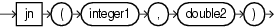Purpose

`jn` is based on `cern.jet.math.Bessel`. It returns the Bessel function of the first kind of order `n` of the argument as a `double`.

This function takes the following arguments:

• `integer1`: the order of the Bessel function `n`.

• `double2`: the value to compute the bessel function of `x`.

For more information, see `http://dsd.lbl.gov/~hoschek/colt/api/cern/jet/math/Bessel.html#jn(int,%20double)`.

Examples

Consider the query `qColt18` in Example 10-97. Given the data stream `SColtFunc` with schema `(c1 integer, c2 double, c3 bigint)` in Example 10-98, the query returns the relation in Example 10-99.

Example 10-97 jn Function Query

```<query id="qColt18"><![CDATA[
select jn(c1,c2) from SColtFunc
]]></query>
```

Example 10-98 jn Function Stream Input

```Timestamp   Tuple
10        1,0.5,8
1000        4,0.7,6
1200        3,0.89,12
2000        8,0.4,4
```

Example 10-99 jn Function Relation Output

```Timestamp   Tuple Kind  Tuple
10:       +           0.24226846
1000:       +           6.1009696E-4
1200:       +           0.0139740035
2000:       +           6.321045E-11
```

## k0

Syntax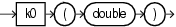Purpose

`k0` is based on `cern.jet.math.Bessel`. It returns the modified Bessel function of the third kind of order 0 of the `double` argument as a `double`.

The range is partitioned into the two intervals `[0,8]` and `(8, infinity)`. Chebyshev polynomial expansions are employed in each interval.

For more information, see `http://dsd.lbl.gov/~hoschek/colt/api/cern/jet/math/Bessel.html#k0(double)`.

Examples

Consider the query `qColt19` in Example 10-100. Given the data stream `SColtFunc` with schema `(c1 integer, c2 double, c3 bigint)` in Example 10-101, the query returns the relation in Example 10-102.

Example 10-100 k0 Function Query

```<query id="qColt19"><![CDATA[
select k0(c2) from SColtFunc
]]></query>
```

Example 10-101 k0 Function Stream Input

```Timestamp   Tuple
10        1,0.5,8
1000        4,0.7,6
1200        3,0.89,12
2000        8,0.4,4
```

Example 10-102 k0 Function Relation Output

```Timestamp   Tuple Kind  Tuple
10:       +           0.92441905
1000:       +           0.6605199
1200:       +           0.49396032
2000:       +           1.1145291
```

## k0e

Syntax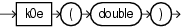Purpose

`k0e` is based on `cern.jet.math.Bessel`. It returns the exponentially scaled modified Bessel function of the third kind of order 0 of the `double` argument as a `double`.

For more information, see `http://dsd.lbl.gov/~hoschek/colt/api/cern/jet/math/Bessel.html#k0e(double)`.

Examples

Consider the query `qColt20` in Example 10-103. Given the data stream `SColtFunc` with schema `(c1 integer, c2 double, c3 bigint)` in Example 10-104, the query returns the relation in Example 10-105.

Example 10-103 k0e Function Query

```<query id="qColt20"><![CDATA[
select k0e(c2) from SColtFunc
]]></query>
```

Example 10-104 k0e Function Stream Input

```Timestamp   Tuple
10        1,0.5,8
1000        4,0.7,6
1200        3,0.89,12
2000        8,0.4,4
```

Example 10-105 k0e Function Relation Output

```Timestamp   Tuple Kind  Tuple
10:       +           1.5241094
1000:       +           1.3301237
1200:       +           1.2028574
2000:       +           1.662682
```

## k1

Syntax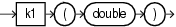Purpose

`k1` is based on `cern.jet.math.Bessel`. It returns the modified Bessel function of the third kind of order 1 of the `double` argument as a `double`.

The range is partitioned into the two intervals `[0,2]` and `(2, infinity)`. Chebyshev polynomial expansions are employed in each interval.

For more information, see `http://dsd.lbl.gov/~hoschek/colt/api/cern/jet/math/Bessel.html#k1(double)`.

Examples

Consider the query `qColt21` in Example 10-106. Given the data stream `SColtFunc` with schema `(c1 integer, c2 double, c3 bigint)` in Example 10-107, the query returns the relation in Example 10-108.

Example 10-106 k1 Function Query

```<query id="qColt21"><![CDATA[
select k1(c2) from SColtFunc
]]></query>
```

Example 10-107 k1 Function Stream Input

```Timestamp   Tuple
10        1,0.5,8
1000        4,0.7,6
1200        3,0.89,12
2000        8,0.4,4
```

Example 10-108 k1 Function Relation Output

```Timestamp   Tuple Kind  Tuple
10:       +           1.6564411
1000:       +           1.0502836
1200:       +           0.7295154
2000:       +           2.1843543
```

## k1e

Syntax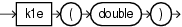Purpose

`k1e` is based on `cern.jet.math.Bessel`. It returns the exponentially scaled modified Bessel function of the third kind of order 1 of the `double` argument as a `double`.

The function is defined as: `k1e(x) = exp(x) * k1(x)`.

• `http://dsd.lbl.gov/~hoschek/colt/api/cern/jet/math/Bessel.html#k1e(double)`

• "k1"

Examples

Consider the query `qColt22` in Example 10-109. Given the data stream `SColtFunc` with schema `(c1 integer, c2 double, c3 bigint)` in Example 10-110, the query returns the relation in Example 10-111.

Example 10-109 k1e Function Query

```<query id="qColt22"><![CDATA[
select k1e(c2) from SColtFunc
]]></query>
```

Example 10-110 k1e Function Stream Input

```Timestamp   Tuple
10        1,0.5,8
1000        4,0.7,6
1200        3,0.89,12
2000        8,0.4,4
```

Example 10-111 k1e Function Relation Output

```Timestamp   Tuple Kind  Tuple
10:       +           2.7310097
1000:       +           2.1150115
1200:       +           1.7764645
2000:       +           3.258674
```

## kn

Syntax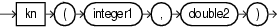Purpose

`kn` is based on `cern.jet.math.Bessel`. It returns the modified Bessel function of the third kind of order `n` of the argument as a `double`.

This function takes the following arguments:

• `integer1`: the `n` value order of the Bessel function.

• `double2`: the `x` value to compute the bessel function of.

The range is partitioned into the two intervals `[0,9.55]` and `(9.55, infinity)`. An ascending power series is used in the low range, and an asymptotic expansion in the high range.

For more information, see `http://dsd.lbl.gov/~hoschek/colt/api/cern/jet/math/Bessel.html#kn(int,%20double)`.

Examples

Consider the query `qColt23` in Example 10-112. Given the data stream `SColtFunc` with schema `(c1 integer, c2 double, c3 bigint)` in Example 10-113, the query returns the relation in Example 10-114.

Example 10-112 kn Function Query

```<query id="qColt23"><![CDATA[
select kn(c1,c2) from SColtFunc
]]></query>
```

Example 10-113 kn Function Stream Input

```Timestamp   Tuple
10        1,0.5,8
1000        4,0.7,6
1200        3,0.89,12
2000        8,0.4,4
```

Example 10-114 kn Function Relation Output

```Timestamp   Tuple Kind  Tuple
10:       +             1.6564411
1000:       +           191.99422
1200:       +            10.317473
2000:       +             9.7876858E8
```

## leastSignificantBit

Syntax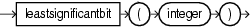Purpose

`leastSignificantBit` is based on `cern.colt.bitvector.QuickBitVector`. It returns the index (as an `integer`) of the least significant bit in state `true` of the `integer` argument. Returns 32 if no bit is in state `true`.

Examples

Consider the query `qColt54` in Example 10-115. Given the data stream `SColtFunc` with schema `(c1 integer, c2 double, c3 bigint)` in Example 10-116, the query returns the relation in Example 10-117.

Example 10-115 leastSignificantBit Function Query

```<query id="qColt54"><![CDATA[
select leastSignificantBit(c1) from SColtFunc
]]></query>
```

Example 10-116 leastSignificantBit Function Stream Input

```Timestamp   Tuple
10        1,0.5,8
1000        4,0.7,6
1200        3,0.89,12
2000        8,0.4,4
```

Example 10-117 leastSignificantBit Function Relation Output

```Timestamp   Tuple Kind  Tuple
10:       +           0
1000:       +           2
1200:       +           0
2000:       +           3
```

## log

Syntax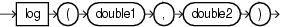Purpose

`log` is based on `cern.jet.math.Arithmetic`. It returns the computation that Figure 10-11 shows as a `double`.

Figure 10-11 cern.jet.math.Arithmetic log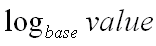This function takes the following arguments:

• `double1`: the `base`.

• `double2`: the `value`.

For more information, see `http://dsd.lbl.gov/~hoschek/colt/api/cern/jet/math/Arithmetic.html#log(double,%20double)`.

Examples

Consider the query `qColt3` in Example 10-118. Given the data stream `SColtFunc` with schema `(c1 integer, c2 double, c3 bigint)` in Example 10-119, the query returns the relation in Example 10-120.

Example 10-118 log Function Query

```<query id="qColt3"><![CDATA[
select log(c2,c2) from SColtFunc
]]></query>
```

Example 10-119 log Function Stream Input

```Timestamp   Tuple
10        1,0.5,8
1000        4,0.7,6
1200        3,0.89,12
2000        8,0.4,4
```

Example 10-120 log Function Relation Output

```Timestamp   Tuple Kind  Tuple
10:       +           1.0
1000:       +           1.0
1200:       +           1.0
2000:       +           1.0
```

## log10

Syntax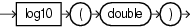Purpose

`log10` is based on `cern.jet.math.Arithmetic`. It returns the base 10 logarithm of a `double` value as a `double`.

For more information, see `http://dsd.lbl.gov/~hoschek/colt/api/cern/jet/math/Arithmetic.html#log10(double)`.

Examples

Consider the query `qColt4` in Example 10-121. Given the data stream `SColtFunc` with schema `(c1 integer, c2 double, c3 bigint)` in Example 10-122, the query returns the relation in Example 10-123.

Example 10-121 log10 Function Query

```<query id="qColt4"><![CDATA[
select log10(c2) from SColtFunc
]]></query>
```

Example 10-122 log10 Function Stream Input

```Timestamp   Tuple
10        1,0.5,8
1000        4,0.7,6
1200        3,0.89,12
2000        8,0.4,4
```

Example 10-123 log10 Function Relation Output

```Timestamp   Tuple Kind  Tuple
10:       +           -0.30103
1000:       +           -0.15490197
1200:       +           -0.050610002
2000:       +           -0.39794
```

## log2

Syntax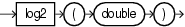Purpose

`log2` is based on `cern.jet.math.Arithmetic`. It returns the base 2 logarithm of a `double` value as a `double`.

For more information, see `http://dsd.lbl.gov/~hoschek/colt/api/cern/jet/math/Arithmetic.html#log2(double)`.

Examples

Consider the query `qColt9` in Example 10-124. Given the data stream `SColtFunc` with schema `(c1 integer, c2 double, c3 bigint)` in Example 10-125, the query returns the relation in Example 10-126.

Example 10-124 log2 Function Query

```<query id="qColt9"><![CDATA[
select log2(c2) from SColtFunc
]]></query>
```

Example 10-125 log2 Function Stream Input

```Timestamp   Tuple
10        1,0.5,8
1000        4,0.7,6
1200        3,0.89,12
2000        8,0.4,4
```

Example 10-126 log2 Function Relation Output

```Timestamp   Tuple Kind  Tuple
10:       +           -1.0
1000:       +           -0.5145732
1200:       +           -0.16812278
2000:       +           -1.321928
```

## logFactorial

Syntax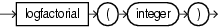Purpose

`logFactorial` is based on `cern.jet.math.Arithmetic`. It returns the natural logarithm (base e) of the factorial of its `integer` argument as a `double`

For argument values `k<30`, the function looks up the result in a table in `O(1)`. For argument values `k>=30`, the function uses Stirlings approximation.

For more information, see `http://dsd.lbl.gov/~hoschek/colt/api/cern/jet/math/Arithmetic.html#logFactorial(int)`.

Examples

Consider the query `qColt10` in Example 10-127. Given the data stream `SColtFunc` with schema `(c1 integer, c2 double, c3 bigint)` in Example 10-128, the query returns the relation in Example 10-129.

Example 10-127 logFactorial Function Query

```<query id="qColt10"><![CDATA[
select logFactorial(c1) from SColtFunc
]]></query>
```

Example 10-128 logFactorial Function Stream Input

```Timestamp   Tuple
10        1,0.5,8
1000        4,0.7,6
1200        3,0.89,12
2000        8,0.4,4
```

Example 10-129 logFactorial Function Relation Output

```Timestamp   Tuple Kind  Tuple
10:       +            0.0
1000:       +            3.1780539
1200:       +            1.7917595
2000:       +           10.604603
```

## logGamma

Syntax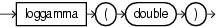Purpose

`logGamma` is based on `cern.jet.stat.Gamma`. It returns the natural logarithm (base e) of the gamma function of the `double` argument as a `double`.

For more information, see `http://dsd.lbl.gov/~hoschek/colt/api/cern/jet/stat/Gamma.html#logGamma(double)`.

Examples

Consider the query `qColt33` in Example 10-130. Given the data stream `SColtFunc` with schema `(c1 integer, c2 double, c3 bigint)` in Example 10-131, the query returns the relation in Example 10-132.

Example 10-130 logGamma Function Query

```<query id="qColt33"><![CDATA[
select logGamma(c2) from SColtFunc
]]></query>
```

Example 10-131 logGamma Function Stream Input

```Timestamp   Tuple
10        1,0.5,8
1000        4,0.7,6
1200        3,0.89,12
2000        8,0.4,4
```

Example 10-132 logGamma Function Relation Output

```Timestamp   Tuple Kind  Tuple
10:       +           0.5723649
1000:       +           0.26086727
1200:       +           0.07402218
2000:       +           0.7966778
```

## longFactorial

Syntax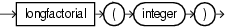Purpose

`longFactorial` is based on `cern.jet.math.Arithmetic`. It returns the factorial of its `integer` argument (in the range `k >= 0 && k < 21`) as a `long`.

For more information, see `http://dsd.lbl.gov/~hoschek/colt/api/cern/jet/math/Arithmetic.html#longFactorial(int)`.

Examples

Consider the query `qColt11` in Example 10-133. Given the data stream `SColtFunc` with schema `(c1 integer, c2 double, c3 bigint)` in Example 10-134, the query returns the relation in Example 10-135.

Example 10-133 longFactorial Function Query

```<query id="qColt11"><![CDATA[
select longFactorial(c1) from SColtFunc
]]></query>
```

Example 10-134 longFactorial Function Stream Input

```Timestamp   Tuple
10        1,0.5,8
1000        4,0.7,6
1200        3,0.89,12
2000        8,0.4,4
```

Example 10-135 longFactorial Function Relation Output

```Timestamp   Tuple Kind  Tuple
10:       +           1
1000:       +           24
1200:       +           6
2000:       +           40320
```

## mostSignificantBit

Syntax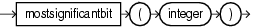Purpose

`mostSignificantBit` is based on `cern.colt.bitvector.QuickBitVector`. It returns the index (as an `integer`) of the most significant bit in state `true` of the `integer` argument. Returns `-1` if no bit is in state `true`

Examples

Consider the query `qColt55` in Example 10-136. Given the data stream `SColtFunc` with schema `(c1 integer, c2 double, c3 bigint)` in Example 10-137, the query returns the relation in Example 10-138.

Example 10-136 mostSignificantBit Function Query

```<query id="qColt55"><![CDATA[
select mostSignificantBit(c1) from SColtFunc
]]></view>
```

Example 10-137 mostSignificantBit Function Stream Input

```Timestamp   Tuple
10        1,0.5,8
1000        4,0.7,6
1200        3,0.89,12
2000        8,0.4,4
```

Example 10-138 mostSignificantBit Function Relation Output

```Timestamp   Tuple Kind  Tuple
10:       +           0
1000:       +           2
1200:       +           1
2000:       +           3
```

## negativeBinomial

Syntax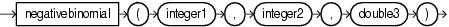Purpose

`negativeBinomial` is based on `cern.jet.stat.Probability`. It returns the sum of the terms 0 through `k` of the Negative Binomial Distribution (see Figure 10-12) as a `double`.

Figure 10-12 cern.jet.stat.Probability negativeBinomial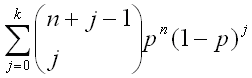This function takes the following arguments:

• `integer1`: the end term `k`.

• `integer2`: the number of trials `n`.

• `double3`: the probability of success `p` in (0.0,1.0).

For more information, see `http://dsd.lbl.gov/~hoschek/colt/api/cern/jet/stat/Probability.html#negativeBinomial(int,%20int,%20double)`.

Examples

Consider the query `qColt44` in Example 10-139. Given the data stream `SColtFunc` with schema `(c1 integer, c2 double, c3 bigint)` in Example 10-140, the query returns the relation in Example 10-141.

Example 10-139 negativeBinomial Function Query

```<query id="qColt44"><![CDATA[
select negativeBinomial(c1,c1,c2) from SColtFunc
]]></query>
```

Example 10-140 negativeBinomial Function Stream Input

```Timestamp   Tuple
10        1,0.5,8
1000        4,0.7,6
1200        3,0.89,12
2000        8,0.4,4
```

Example 10-141 negativeBinomial Function Relation Output

```Timestamp   Tuple Kind  Tuple
10:       +           0.75
1000:       +           0.94203234
1200:       +           0.99817264
2000:       +           0.28393665
```

## negativeBinomialComplemented

Syntax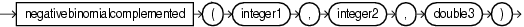Purpose

`negativeBinomialComplemented` is based on `cern.jet.stat.Probability`. It returns the sum of the terms `k+1` to infinity of the Negative Binomial distribution (see Figure 10-13) as a `double`.

Figure 10-13 cern.jet.stat.Probability negativeBinomialComplemented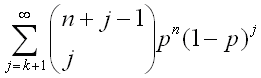This function takes the following arguments:

• `integer1`: the end term `k`.

• `integer2`: the number of trials `n`.

• `double3`: the probability of success `p` in (0.0,1.0).

For more information, see `http://dsd.lbl.gov/~hoschek/colt/api/cern/jet/stat/Probability.html#negativeBinomialComplemented(int,%20int,%20double)`.

Examples

Consider the query `qColt45` in Example 10-142. Given the data stream `SColtFunc` with schema `(c1 integer, c2 double, c3 bigint)` in Example 10-143, the query returns the relation in Example 10-144.

Example 10-142 negativeBinomialComplemented Function Query

```<query id="qColt45"><![CDATA[
select negativeBinomialComplemented(c1,c1,c2) from SColtFunc
]]></query>
```

Example 10-143 negativeBinomialComplemented Function Stream Input

```Timestamp   Tuple
10        1,0.5,8
1000        4,0.7,6
1200        3,0.89,12
2000        8,0.4,4
```

Example 10-144 negativeBinomialComplemented Function Relation Output

```Timestamp   Tuple Kind  Tuple
10:       +           0.25
1000:       +           0.05796766
1200:       +           0.0018273441
2000:       +           0.7160633
```

## normal

Syntax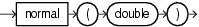Purpose

`normal` is based on `cern.jet.stat.Probability`. It returns the area under the Normal (Gaussian) probability density function, integrated from minus infinity to the `double` argument `x` (see Figure 10-14) as a `double`.

Figure 10-14 cern.jet.stat.Probability normalFor more information, see `http://dsd.lbl.gov/~hoschek/colt/api/cern/jet/stat/Probability.html#normal(double)`.

Examples

Consider the query `qColt46` in Example 10-145. Given the data stream `SColtFunc` with schema `(c1 integer, c2 double, c3 bigint)` in Example 10-146, the query returns the relation in Example 10-147.

Example 10-145 normal Function Query

```<query id="qColt46"><![CDATA[
select normal(c2) from SColtFunc
]]></query>
```

Example 10-146 normal Function Stream Input

```Timestamp   Tuple
10        1,0.5,8
1000        4,0.7,6
1200        3,0.89,12
2000        8,0.4,4
```

Example 10-147 normal Function Relation Output

```Timestamp   Tuple Kind  Tuple
10:       +           0.69146246
1000:       +           0.7580363
1200:       +           0.81326705
2000:       +           0.65542173
```

## normal1

Syntax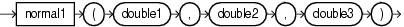Purpose

`normal1` is based on `cern.jet.stat.Probability`. It returns the area under the Normal (Gaussian) probability density function, integrated from minus infinity to `x` (see Figure 10-15) as a `double`.

Figure 10-15 cern.jet.stat.Probability normal1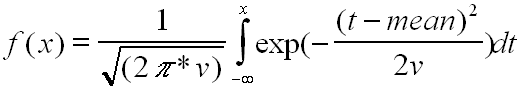This function takes the following arguments:

• `double1`: the normal distribution `mean`.

• `double2`: the variance of the normal distribution `v`.

• `double3`: the integration limit `x`.

For more information, see `http://dsd.lbl.gov/~hoschek/colt/api/cern/jet/stat/Probability.html#normal(double,%20double,%20double)`.

Examples

Consider the query `qColt47` in Example 10-148. Given the data stream `SColtFunc` with schema `(c1 integer, c2 double, c3 bigint)` in Example 10-149, the query returns the relation in Example 10-150.

Example 10-148 normal1 Function Query

```<query id="qColt47"><![CDATA[
select normal1(c2,c2,c2) from SColtFunc
]]></query>
```

Example 10-149 normal1 Function Stream Input

```Timestamp   Tuple
10        1,0.5,8
1000        4,0.7,6
1200        3,0.89,12
2000        8,0.4,4
```

Example 10-150 normal1 Function Relation Output

```Timestamp   Tuple Kind  Tuple
10:       +           0.5
1000:       +           0.5
1200:       +           0.5
2000:       +           0.5
```

## normalInverse

Syntax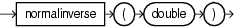Purpose

`normalInverse` is based on `cern.jet.stat.Probability`. It returns the `double` value, `x`, for which the area under the Normal (Gaussian) probability density function (integrated from minus infinity to `x`) equals the `double` argument `y` (assumes mean is zero and variance is one).

For more information, see `http://dsd.lbl.gov/~hoschek/colt/api/cern/jet/stat/Probability.html#normalInverse(double)`.

Examples

Consider the query `qColt48` in Example 10-151. Given the data stream `SColtFunc` with schema `(c1 integer, c2 double, c3 bigint)` in Example 10-152, the query returns the relation in Example 10-153.

Example 10-151 normalInverse Function Query

```<query id="qColt48"><![CDATA[
select normalInverse(c2) from SColtFunc
]]></view>
```

Example 10-152 normalInverse Function Stream Input

```Timestamp   Tuple
10        1,0.5,8
1000        4,0.7,6
1200        3,0.89,12
2000        8,0.4,4
```

Example 10-153 normalInverse Function Relation Output

```Timestamp   Tuple Kind  Tuple
10:       +           0.0
1000:       +           0.5244005
1200:       +           1.226528
2000:       +           0.2533471
```

## poisson

Syntax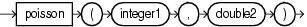Purpose

`poisson` is based on `cern.jet.stat.Probability`. It returns the sum of the first `k` terms of the Poisson distribution (see Figure 10-16) as a `double`.

Figure 10-16 cern.jet.stat.Probability poisson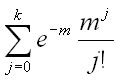This function takes the following arguments:

• `integer1`: the number of terms `k`.

• `double2`: the mean of the Poisson distribution `m`.

For more information, see `http://dsd.lbl.gov/~hoschek/colt/api/cern/jet/stat/Probability.html#poisson(int,%20double)`.

Examples

Consider the query `qColt49` in Example 10-154. Given the data stream `SColtFunc` with schema `(c1 integer, c2 double, c3 bigint)` in Example 10-155, the query returns the relation in Example 10-156.

Example 10-154 poisson Function Query

```<query id="qColt49"><![CDATA[
select poisson(c1,c2) from SColtFunc
]]></query>
```

Example 10-155 poisson Function Stream Input

```Timestamp   Tuple
10        1,0.5,8
1000        4,0.7,6
1200        3,0.89,12
2000        8,0.4,4
```

Example 10-156 poisson Function Relation Output

```Timestamp   Tuple Kind  Tuple
10:       +           0.909796
1000:       +           0.9992145
1200:       +           0.9870295
2000:       +           1.0
```

## poissonComplemented

Syntax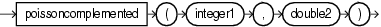Purpose

`poissonComplemented` is based on `cern.jet.stat.Probability`. It returns the sum of the terms `k+1` to Infinity of the Poisson distribution (see Figure 10-17) as a `double`.

Figure 10-17 cern.jet.stat.Probability poissonComplemented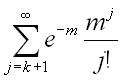This function takes the following arguments:

• `integer1`: the start term `k`.

• `double2`: the mean of the Poisson distribution `m`.

For more information, see `http://dsd.lbl.gov/~hoschek/colt/api/cern/jet/stat/Probability.html#poissonComplemented(int,%20double)`.

Examples

Consider the query `qColt50` in Example 10-157. Given the data stream `SColtFunc` with schema `(c1 integer, c2 double, c3 bigint)` in Example 10-158, the query returns the relation in Example 10-159.

Example 10-157 poissonComplemented Function Query

```<query id="qColt50"><![CDATA[
select poissonComplemented(c1,c2) from SColtFunc
]]></query>
```

Example 10-158 poissonComplemented Function Stream Input

```Timestamp   Tuple
10        1,0.5,8
1000        4,0.7,6
1200        3,0.89,12
2000        8,0.4,4
```

Example 10-159 poissonComplemented Function Relation Output

```Timestamp   Tuple Kind  Tuple
10:       +           0.09020401
1000:       +           7.855354E-4
1200:       +           0.012970487
2000:       +           5.043364E-10
```

## stirlingCorrection

Syntax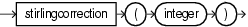Purpose

`stirlingCorrection` is based on `cern.jet.math.Arithmetic`. It returns the correction term of the Stirling approximation of the natural logarithm (base e) of the factorial of the `integer` argument (see Figure 10-18) as a `double`.

Figure 10-18 cern.jet.math.Arithmetic stirlingCorrection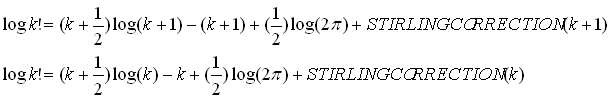For more information, see `http://dsd.lbl.gov/~hoschek/colt/api/cern/jet/math/Arithmetic.html#stirlingCorrection(int)`.

Examples

Consider the query `qColt5` in Example 10-160. Given the data stream `SColtFunc` with schema `(c1 integer, c2 double, c3 bigint)` in Example 10-161, the query returns the relation in Example 10-162.

Example 10-160 stirlingCorrection Function Query

```<query id="qColt5"><![CDATA[
select stirlingCorrection(c1) from SColtFunc
]]></query>
```

Example 10-161 stirlingCorrection Function Stream Input

```Timestamp   Tuple
10        1,0.5,8
1000        4,0.7,6
1200        3,0.89,12
2000        8,0.4,4
```

Example 10-162 stirlingCorrection Function Relation Output

```Timestamp   Tuple Kind  Tuple
10:       +           0.08106147
1000:       +           0.020790672
1200:       +           0.027677925
2000:       +           0.010411265
```

## studentT

Syntax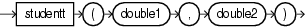Purpose

`studentT` is based on `cern.jet.stat.Probability`. It returns the integral from minus infinity to `t` of the Student-t distribution with `k` > 0 degrees of freedom (see Figure 10-19) as a `double`.

Figure 10-19 cern.jet.stat.Probability studentT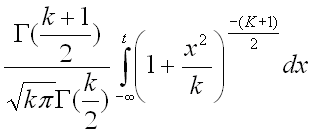This function takes the following arguments:

• `double1`: the degrees of freedom `k`.

• `double2`: the integration end point `t`.

For more information, see `http://dsd.lbl.gov/~hoschek/colt/api/cern/jet/stat/Probability.html#studentT(double,%20double)`.

Examples

Consider the query `qColt51` in Example 10-163. Given the data stream `SColtFunc` with schema `(c1 integer, c2 double, c3 bigint)` in Example 10-164, the query returns the relation in Example 10-165.

Example 10-163 studentT Function Query

```<query id="qColt51"><![CDATA[
select studentT(c2,c2) from SColtFunc
]]></query>
```

Example 10-164 studentT Function Stream Input

```Timestamp   Tuple
10        1,0.5,8
1000        4,0.7,6
1200        3,0.89,12
2000        8,0.4,4
```

Example 10-165 studentT Function Relation Output

```Timestamp   Tuple Kind  Tuple
10:       +           0.621341
1000:       +           0.67624015
1200:       +           0.7243568
2000:       +           0.5930112
```

## studentTInverse

Syntax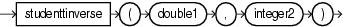Purpose

`studentTInverse` is based on `cern.jet.stat.Probability`. It returns the `double` value, `t`, for which the area under the Student-t probability density function (integrated from minus infinity to `t`) equals `1-alpha/2`. The value returned corresponds to the usual Student t-distribution lookup table for `talpha[size]`. This function uses the `studentt` function to determine the return value iteratively.

This function takes the following arguments:

• `double1`: the probability `alpha`.

• `integer2`: the data set size.

• `http://dsd.lbl.gov/~hoschek/colt/api/cern/jet/stat/Probability.html#studentTInverse(double,%20int)`

• "studentT"

Examples

Consider the query `qColt52` in Example 10-166. Given the data stream `SColtFunc` with schema `(c1 integer, c2 double, c3 bigint)` in Example 10-167, the query returns the relation in Example 10-168.

Example 10-166 studentTInverse Function Query

```<query id="qColt52"><![CDATA[
select studentTInverse(c2,c1) from SColtFunc
]]></query>
```

Example 10-167 studentTInverse Function Stream Input

```Timestamp   Tuple
10        1,0.5,8
1000        4,0.7,6
1200        3,0.89,12
2000        8,0.4,4
```

Example 10-168 studentTInverse Function Relation Output

```Timestamp   Tuple Kind  Tuple
10:       +           1.0
1000:       +           0.4141633
1200:       +           0.15038916
2000:       +           0.8888911
```

## y0

Syntax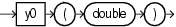Purpose

`y0` is based on `cern.jet.math.Bessel`. It returns the Bessel function of the second kind of order 0 of the `double` argument as a `double`.

For more information, see `http://dsd.lbl.gov/~hoschek/colt/api/cern/jet/math/Bessel.html#y0(double)`.

Examples

Consider the query `qColt24` in Example 10-169. Given the data stream `SColtFunc` with schema `(c1 integer, c2 double, c3 bigint)` in Example 10-170, the query returns the relation in Example 10-171.

Example 10-169 y0 Function Query

```<query id="qColt24"><![CDATA[
select y0(c2) from SColtFunc
]]></query>
```

Example 10-170 y0 Function Stream Input

```Timestamp   Tuple
10        1,0.5,8
1000        4,0.7,6
1200        3,0.89,12
2000        8,0.4,4
```

Example 10-171 y0 Function Relation Output

```Timestamp   Tuple Kind  Tuple
10:       +           -0.44451874
1000:       +           -0.19066493
1200:       +           -0.0031519707
2000:       +           -0.60602456
```

## y1

Syntax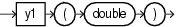Purpose

`y1` is based on `cern.jet.math.Bessel`. It returns the Bessel function of the second kind of order 1 of the float argument as a `double`.

For more information, see `http://dsd.lbl.gov/~hoschek/colt/api/cern/jet/math/Bessel.html#y1(double)`.

Examples

Consider the query `qColt25` in Example 10-172. Given the data stream `SColtFunc` with schema `(c1 integer, c2 double, c3 bigint)` in Example 10-173, the query returns the relation in Example 10-174.

Example 10-172 y1 Function Query

```<query id="qColt25"><![CDATA[
select y1(c2) from SColtFunc
]]></query>
```

Example 10-173 y1 Function Stream Input

```Timestamp   Tuple
10        1,0.5,8
1000        4,0.7,6
1200        3,0.89,12
2000        8,0.4,4
```

Example 10-174 y1 Function Relation Output

```Timestamp   Tuple Kind  Tuple
10:       +           -1.4714724
1000:       +           -1.1032499
1200:       +           -0.88294965
2000:       +           -1.780872
```

## yn

Syntax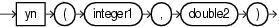Purpose

`yn` is based on `cern.jet.math.Bessel`. It returns the Bessel function of the second kind of order `n` of the `double` argument as a `double`.

This function takes the following arguments:

• `integer1`: the `n` value order of the Bessel function.

• `double2`: the `x` value to compute the Bessel function of.

For more information, see `http://dsd.lbl.gov/~hoschek/colt/api/cern/jet/math/Bessel.html#yn(int,%20double)`.

Examples

Consider the query `qColt26` in Example 10-175. Given the data stream `SColtFunc` with schema `(c1 integer, c2 double, c3 bigint)` in Example 10-176, the query returns the relation in Example 10-177.

Example 10-175 yn Function Query

```<query id="qColt26"><![CDATA[
select yn(c1,c2) from SColtFunc
]]></query>
```

Example 10-176 yn Function Stream Input

```Timestamp   Tuple
10        1,0.5,8
1000        4,0.7,6
1200        3,0.89,12
2000        8,0.4,4
```

Example 10-177 yn Function Relation Output

```Timestamp   Tuple Kind  Tuple
10:       +             -1.4714724
1000:       +           -132.63406
1200:       +             -8.020442
2000:       +             -6.3026547E8
```